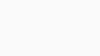### Glossary of Time Value of Money Terms

Michael Sack Elmaleh , CPA, CVA###### Video: Compound Interest

Let’s begin with interest calculations. Let’s suppose you open a savings account, and you deposit \$5,000 today. We call this \$5,000 the principal or present value (PV). The compounding rate or period defines how frequently the bank will credit your account for interest earned. In the formulas that follow, and on most calculators, this compounding period is represented by the letter “n”.

The interest rate per compounding period is usually abbreviated by the letter “i”. Sometimes it is represented by the “%” symbol. Usually, you have to enter the interest rate per compounding period. So, for example if your annual interest rate is 5% and you have four compounding periods per year you would enter 1.25% into your calculator.

###### Simple vs. Compound Interest

There are two methods by which a bank might pay you interest: simple and compound. The simple  method is based only on your original \$5,000 deposit.

The more typical way banks credit interest is the compound interest method. In this method, interest is payed on the original principal plus the previously payed interest. You earn interest on the previously earned interest. This compounding of course assumes that you have not withdrawn the previously payed interest earned.

The table below illustrates both simple and compound interest computations assuming an interest rate of 5% compounded annually. So, starting with \$5,000 today we would accumulate \$6,381 by the end of year five under the compounding method versus \$6,250 or about \$131 less using the simple interest approach.

###### Nominal vs. Effective Interest

Now almost all computations of interest involving financial instruments and accounts utilize compounding computations. So, it is important to note the difference between what is called nominal versus effective interest rates. Usually, a bank will advertise both a nominal interest rate and an effective interest rate. A nominal rate is usually a rate assuming one annual compounding period. But most banks and other financial institutions will compound interest more frequently. The relationship between the nominal and effective interest rate is described by this formula:

The table below shows the relationship between the nominal and effective interest rate holding the number of compounding periods constant at 12. You can see that the effective interest increases with increasing nominal interest rate.

###### Euler’s Constant Emerges

Now a very interesting pattern emerges when a nominal interest rate is held constant and we greatly increase the number of compounding periods, not just within one year but over several years. In the table below we assume \$1 is put in a bank account and will earn 5% nominal interest over 20 years. In the last column you begin to see the accumulated balance get closer and closer to a very important number known as “e”, or Euler’s constant. As the compounding periods increase the accumulated balance will get closer and closer to 2.71828 which is e to six significant digits. This constant appears in many important applications particularly in probability and statistics.

###### Future Values

Finally, the last column of this table contains the general compound interest formula for computing the future value of a lump sum. This lump sum we have been calling the present value or initial deposit. The accumulated balance we will refer to as the future value.

Again, the above formula and example assumes that all interest is reinvested or compounded. Importantly, the above formula allows us to convert one lump sum payment in the future to an equivalent present value and vice versa. By rearranging terms, we arrive at the formula for converting a future value into a present value

Now these formulas can be expanded to cover cases of multiple future payments and a short cut can be applied if the future payments are equal. If we apply the above PV formula to each payment, we can see how this works:

Now there is no need to memorize these formulas because even the simplest of calculators and every spreadsheet program has built in functions that will perform the computations. You just need to be careful to apply the functions in the right order. You also need to know when to use these formulas…and when not to. All these formulas are extremely sensitive to the choice of interest rates so perhaps the most important question to be answered is ‘how do we choose an appropriate interest rate?”. Before continuing with the mechanics and application of present value and compound interest formulas in the next link I want to discuss how interest rates are established